# Fillet welds tensile tested in beam test

### The test in pictures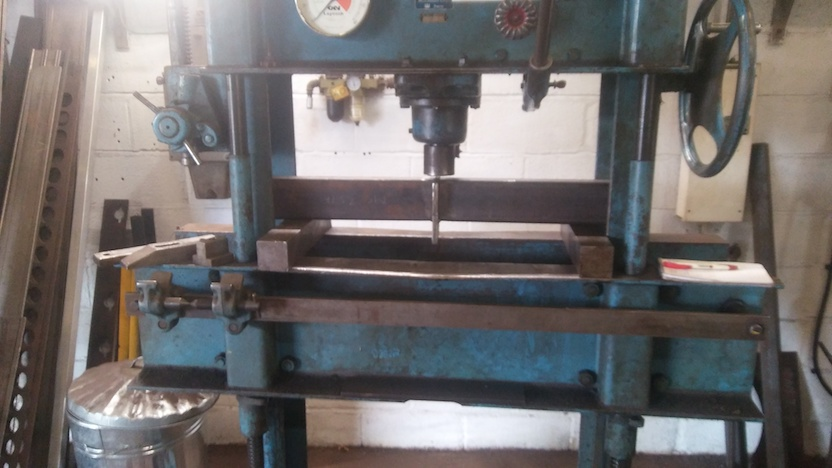First try. Bearers here at 600mm separation - not the 320mm of the test.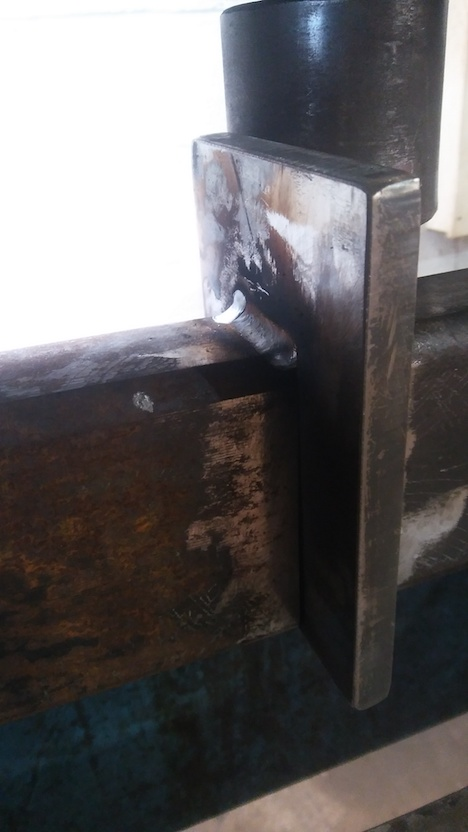Test sample beam upside-down, showing one T-fillet weld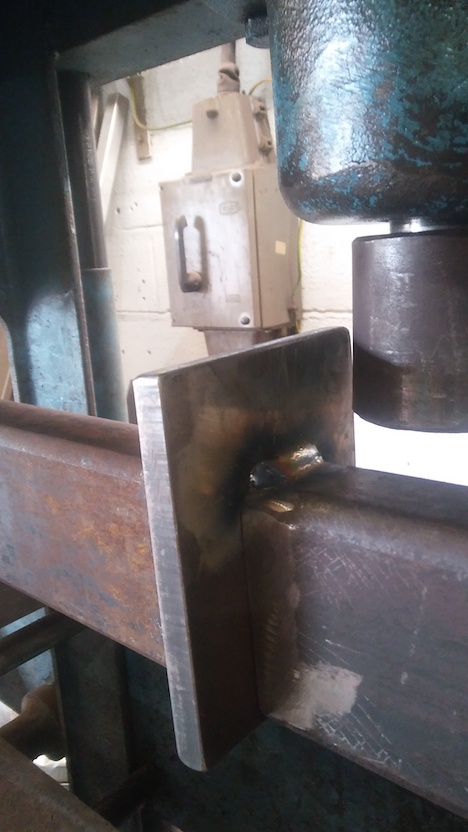... and the T-fillet weld on the opposite side of the central plate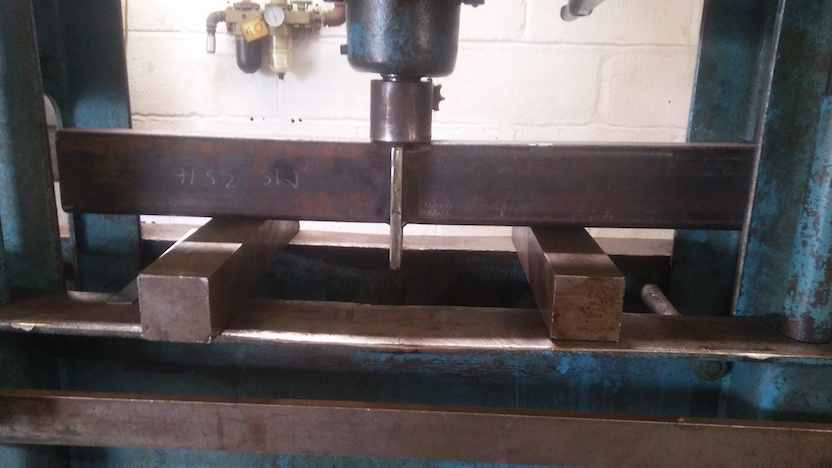The test - press, bearers and beam weld sample set-up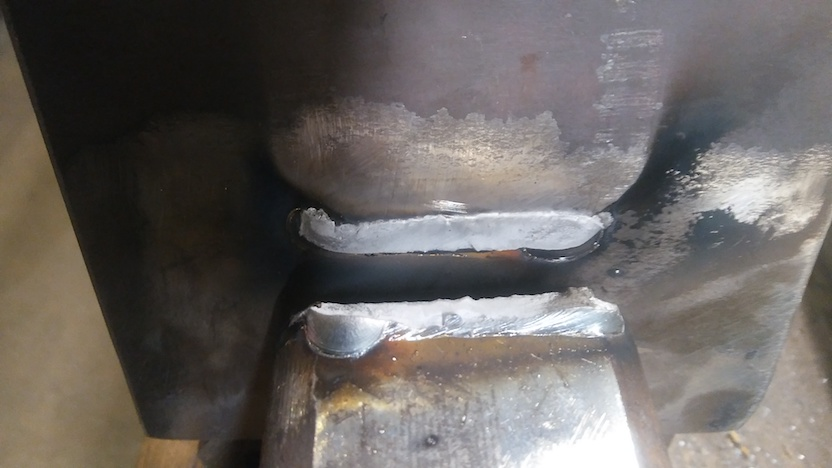The fracture surface(s). Near beam presented upside-down; plate presented "correct way up" (in orientation as put in the press for testing). Fracture is almost planar and is a very fine dimpled apparently ductile "microvoid coalescence" fracture judged by characteristic matt-grey appearance. The fracture is seemingly at or near the weld-metal to parent-plate (the RHS) fusion boundary.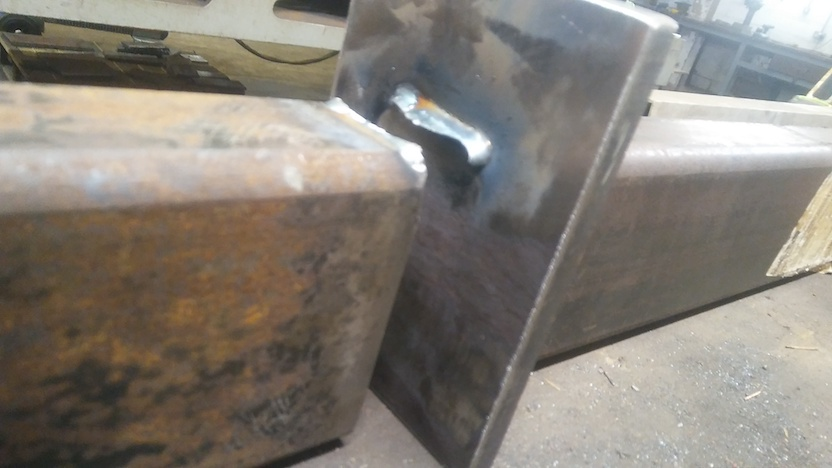Separated halves of the sample presented "as they match", but upside-down to show the fractured weld

### Details about the test

• Weld - GMAW - "spray" 240A (7.5m/min of 1.2mm wire) at 28.5V
• G3Si1 wire
• 6mm leg-length fillets ("z6")
• Fillet welds 40mm long
• 320mm between supports - the bearers
• 18Tonnes-force on gauge at breakage of sample

Breakage was abrupt with loud "bang" and elastic spring of parted sample halves.

### Note at time of test

Note even as the test showed itself as qualitatively interesting and possibly useful...

Overall - test method is a resounding (!!) success, even as it stands now.

## Stresses in weld analysed

### Our "way in" - Static system - Newton's Laws - "sum to zero"

The test, probably best visualised as seen in the two photographs showing the overall test, is a static system. After pumping the press, the forces settle to a static equilibrium. This means the nett sum of forces must be zero. Without losing sight of the overall generality: the sum of (linear) forces is zero and the sum of moments ("twists", "torques") is zero.

This easily visualised mechanical system remains so right up to the breaking point. Whereupon the pieces of the test sample do "develop motion" / "cease to be static" (!).

This fundamental basic Newtonian insight of "sum to zero" is our way in to obtaining the force in the weld in relation to the force the press piston is exerting. This will almost certainly be a in a simple ratio - and that proves to be the case.

The "sum of forces = 0" is easy to see, but is "tautological" and doesn't enable us to deduce anything. You can see that, with a system symmetrical about the press piston centre, the two bearers each resist the press-piston force with half that force vertically upwards by each bearer upon the sample ends.

In the derivation I am about to present, I have already seen this and jumped straight to the system of Moments.

### My derivation...

This is what the beam with fillet welds applies "in return", in satisfying Newton's Laws - "sum to zero" - particularly obviously for a static system.

The press is applying a force with its piston - the value shown on the "F=P.A estimate" force gauge. So the test piece must be identically resisting in applying that same force back upon the piston - Newton's equal-and-opposite ("equal but opposite") force...

This is what the press is applying: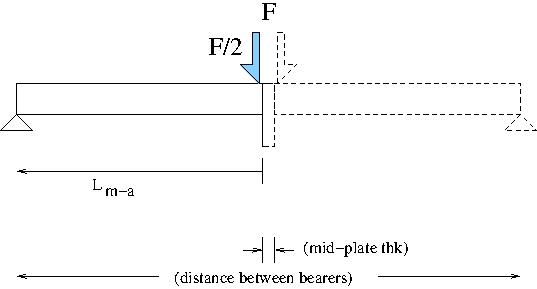(PDF graphic)

which is the "M_s = F/2*L_m-a" applied Moment (see derivation following). This "applied" Moment is "clockwise".

This is what the test-beam must be reacting where the weld attaches the RHS to the central "mid" plate: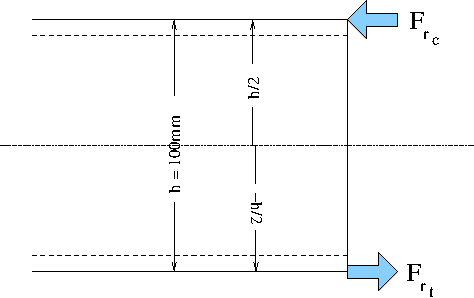(PDF graphic)

which is the "M_r = F_r_t.h/2+F_r_c.-h/2" equal-but-opposite reacted Moment (see derivation following). This "reacted" Moment is "anti-clockwise".

That these two Moments, the applied and the reacted, must be equal but opposite (clockwise and anticlockwise), in order to have a system which is doing nothing when equilibriated ("settled down") to the load applied by the pumped-up press, is the basis of the derivation following.
This logical statement appears mathematically in the line "M_s = - M_r".

Symbols used in developing the derivation:

• M_s = Moment applied by the system
• M_r = Moment reacted by the test-sample
• F_p = Force applied by the press piston
• L_m-a = Length of the moment-arm
• F_r_t = Force of reaction in tension across the weld
• F_r_c = Force of reaction in compression at the opposite side of the section forming the test sample
• h = height of the RHS - which is 100mm for this 100x50(x8) section

By Newton's Laws
M_s = - M_r
which is the fundamental underpinning of this analysis.

I derive for each "half system" - given two identical back-to-back systems about the press-piston central vertical axis...

applied Moment for "system"

M_s=F_p/2*L_m-a
v
system
press
moment-arm

for the reaction - symmetrical about section centre ("the neutral axis")

M_r=F_r_t.h/2+F_r_c.-h/2
F_r_t=-F_r_c
(previous two lines / statements - that must be so - instinct says that - proof not visualised; symmetry about the RHS's longitudinal-horizontal centre being the basis of the instinct about the equilibrium)
M_r=F_r_t.h/2-F_r_c.h/2
M_r=F_r_t.h/2+F_r_t.h/2
M_r=F_r_t.h

combining the applied and the reaction (guided by Newton's Law(s))

F_p/2*L_m-a=-F_r_t.h

the "minus" will signify that the press is applying a downward force while the sample with weld is reacting with an upward force

-F_r_t.h=F_p/2*L_m-a
F_r_t.h=-F_p/2*L_m-a
F_r_t=-F_p*L_m-a/2h

"L_m-a/2h" is a dimensionless ratio

Restating and expanding the comments:

• F_r_t --> what we want - the tensile force with which weld is reacting to oppose the press piston force
• F_p is the force applied by the press piston - which we know from the press's force-gauge
• L_m-a/2h is what we hoped for - a constant value, the ratio of the test's moment-arm to the section's height - with the "2" on the denominator coming from there being identical "left-hand-side" and "right-hand-side" halves of the test sample as a beam sharing the load - clear, simple and excellent

As the force across the weld F_w = F_r_t,

F_w=-F_p*L_m-a/2h

ignoring signifying direction of force, in mathematical notation

|F_w|=|F_p|*L_m-a/2h

So we have exactly what we hoped would "drop-out" if we worked the derivation.
The simplicity means, on a practical level, that we can freely vary the bearer position to make the weld breaking force higher or lower at-will, so that breaking force is somewhere in the mid-range of the press's ability. Not too high for the press to break the sample (bearers too close together); not too low that you are trying to judge a tiny force reading (bearers too far apart).
Bonus - as "L_m-a/2h" is a dimensionless ratio, we don't even have to "watch" our distance units - we can measure in whatever familiar units we want - in a workshop typically in millimetres - so long as we use the same units for bearer-spacing and section-height :-)
This is all totally fantastic out-turn of events!

### Getting quantitative answer for the weld

Now to input numbers / values.

I've done my maths "inline" with the text-editor's lisp ability, injecting the answer into this buffer being worked upon.
That means if you want to read my maths, you will have to adjust to the "lisp style" operator prefix notation (rather than the familiar "on-paper" "infix" notation), between the brackets denoting each lisp "sexp" ("s-expression"). Then there's one or two other quirks from getting the lisp interpreter to give what is physically meant here.
";" is the symbol for "a comment" in lisp (what's to the right-h-s of this the computer ignores) - which I've made syntactic use of with ";;"'s and also breaking up statements for the human reading.
There's some "infix notation" for the human developing the maths, and some prefix notation for the lisp expression executed in-line here.

L_m-a/2h=...

Some measurements which are the input values to this arithmetic:

• 320mm = the distance bearers are set apart
• 12mm = the thickness of the steel plate inserted at the middle of the sample between the RHS section ends, enabling the test T-fillet welds to be deposited
• 18 Tonnes-force = the force shown on the press's force-gauge when the sample broken through one of the test welds

L_m-a=(320-12)/2 ;; (/ (- 320 12) 2e0) ;; 154.0
L_m-a/2h=154/2*100=154/200= ;; (/ 154 200e0) ;; 0.77

So there we have it,
"L_m-a/2h" = 0.77
for this test set-up with a 100mm tall RHS and 320mm between the bearers.

F_w=
(*
(* 18 1000 9.81) ;; 176580.0 ;; force the press is exerting (N)
0.77 ;; the geometric ratio for this set-up
) ;; 135966.6 ;; N

or another way to put it
(* 18 0.77) ;; 13.86 ;; Tonnes-force

So we have already deduced that each test weld is reacting with 13.86 Tonnes-force tensile at the instant one of the welds broke.

Onwards - the weld - 6mm leg-length.
When I welded it, I made that so.
In standard weld terminology, the leg-length (fillet size measured along the "plate" surface - the "face" / "edge" of the triangular shape - is given the symbol "z". Such that we could denote the size of the fillet weld as "z6".

More symbols

• z = fillet leg-length - as just explained
• L_z == "z"
• L_w = the length of the weld (is 40mm here)
• sigma (we'd like to use the Greek letter "sigma" to denote this) is the stress (Force per unit area) - SI unit Pa - typically expressed in MPa (mega-Pascals)
• sigma_f_z = the stress in the fillet weld(s) along the "z" direction - parallel to the length of the RHS half-beam surface, at the interface between the fillet weld and the RHS surface
• throat - no symbol used (conventionally "a" in weld terminology) - is the "short" distance through the diagonal of the weld's triangular shape

and more measurements (some restated)

• 6mm = the fillet size as "leg length"
• 40mm = the length of the test T-fillet welds (across the 50mm width of the RHS's)

sigma_f-z=F_w/(L_z.L_w)
(*
(/ 135966.6 (* 6e-3 40e-3)) ;; 566527500.0
1e-6) ;; 566.5274999999999 ;; MPa

That is a notable value - which deserves significant comment - which will come a bit later...

### arithmetic - onwards...

What if consider shear (Wikipedia) along the throat?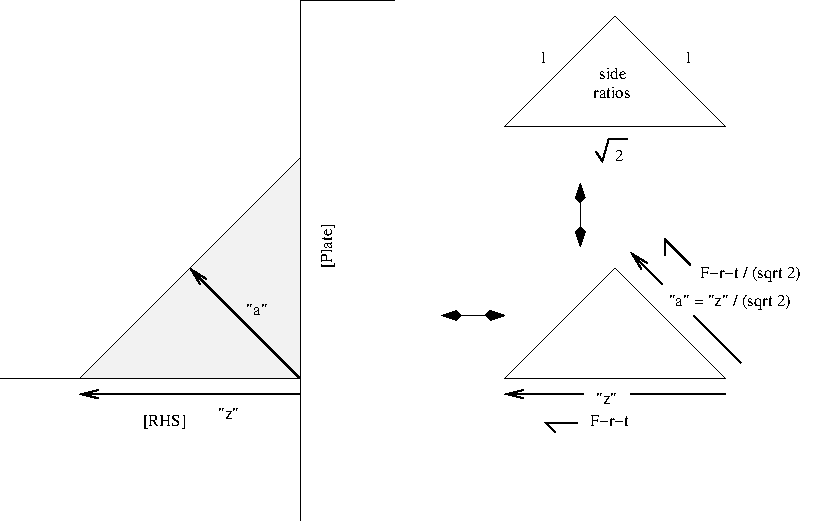(PDF graphic)

Noting F_w = F_r_t,

cos45=(F_w/2)/s

• s=shear - where one part of an object tends to or does slide across another part of the object under a shearing force

s=F_w/2cos45

(/
135966.6 ;; N
(* 2 (cosd 45)) ;; 1.4142135623730951
) ;; 96142.90487487883 N

the (sqrt 2)/2 on F_w and on L_z cross-cancel - likely has equal shear stress on any plane between the longi-leg and the throat (??)

Little demonstration - "cos45" and "root-2/2" are equal,
given "=" is a question, a test, for equality in emacs (the text-editor's) lisp
(= (cosd 45) (/ (sqrt 2) 2)) ;; t
where the answer "t" means "true" (anything that is not "t" is "nil")

here it is...
(/
(* 135966.6 (cosd 45)) ;; 96142.90487487885
(* 6e-3 (cosd 45) 40e-3)) ;; 566527500.0

which,
disregarding sub-negligible "floating-point error" on the computed values output by the emacs lisp interpreter,
is one and the same as the calculated stress along the fillet boundary between weld metal and the "parent" steel of the RHS.

### arithmetic - calculated weld strength - comment

567MPa - that is a familiar break stress for a weld.

I can't be "leading" on this - see data-sheets for "G3Si1" / "ER70s-6" GMAW / MIG wire from the major welding consumable manufacturers - Esab, Lincoln Electric, etc - plus any others you may choose to look at.

Basically - 560MPa is the expected breaking strength (ultimate tensile strength) of "G3Si1" weld metal.

[07Feb2021 note - don't jump to conclusions yet - the "S355" steel constrained to not plastically deform might behave under load in ways not producing familiar numbers - with systematic reasons to suspect the "S355" steel in these circumstances might abruptly fracture at around the 560MPa of weld metal break]

My calculated value "is too good to be true".
That has the look of

• scoring a "bullseye" on a 600metre rifle range - with a smooth-bore flintlock pistol
• scoring a goal at the World Cup final by a player wearing a steamed-up diving-mask and lead-weighted diver's boots

On that note and at that juncture, I will leave the quantitative analysis as "to be continued..." - were there to be more data to evaluate.

The problem is - this can't be real. If it were that easy to obtain the weld strength of a fillet weld, it would already be done that way. You can know this from competitiveness to get the best results cheapest, which gives very reliable deductions where there are many participants acting independently.
Uniaxial tensile testing machines big enough to test meaningful fillet weld samples have to be able to apply at least 100Tonnes-force - which make them enormous beasts, well above the size of a commercial test-house's "tensile testing machine".

Something I was genuinely completely unsure about - there seemed the possibility that the weld strength in an apparent shear loading would calculate as being about half the weld's tensile strength. It is a familiar result via arcane arguments that the shear yield is half the uniaxial tensile yield.
I need to consult on that.

Given all considerations...

I'm leaving things at that, feeling rather bemused.

(R. Smith, 24Nov2020, 28Nov2020 (added maths analysis), 05Dec2020 (added analytical derivation figs), 06Dec2020 (clock anti-clock), 07Feb2021 (F_r_t=F_w, aname))